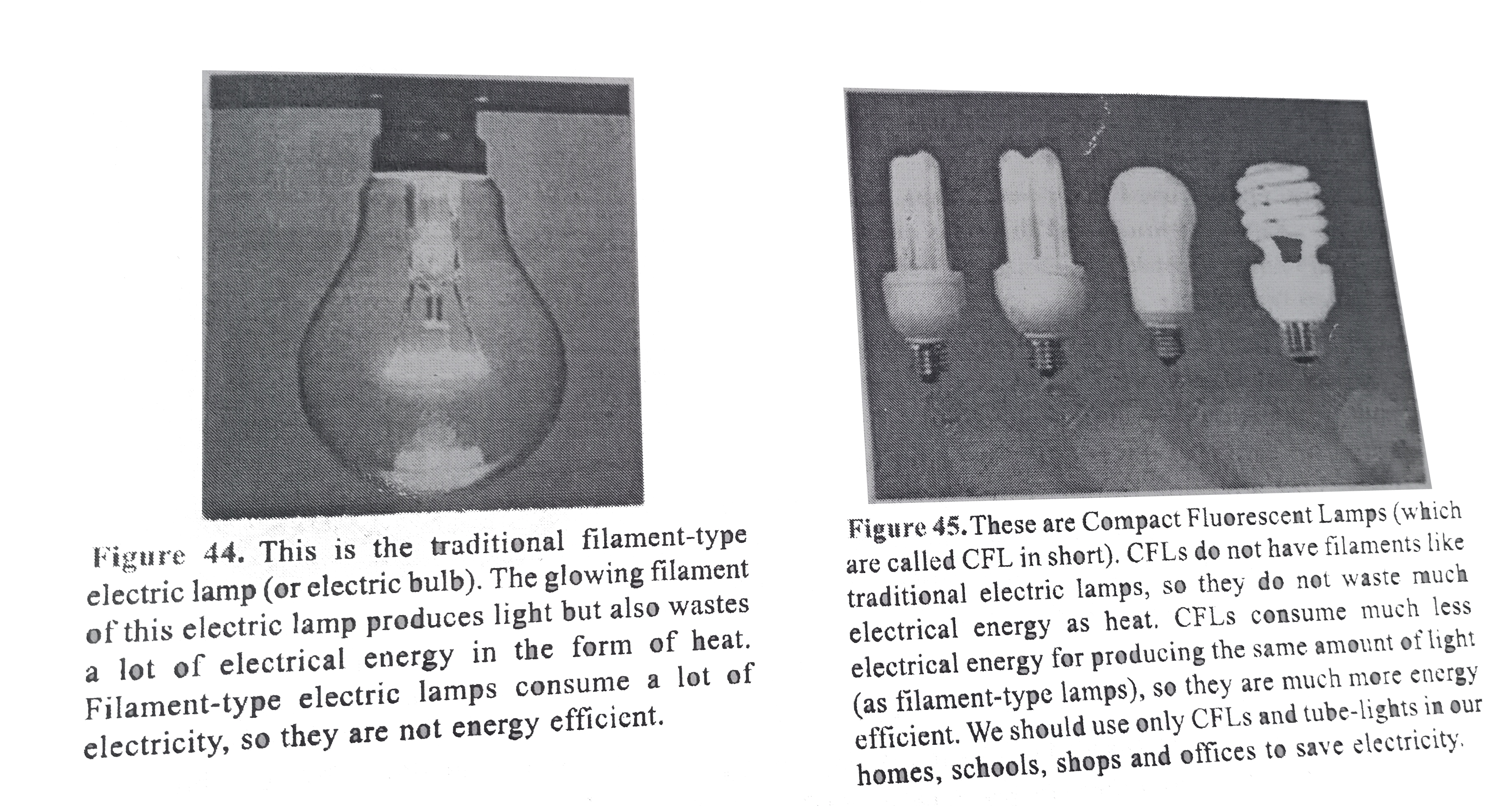Question

Updated on:26/04/2023

# An electric bulb consumes 7.2 kJ of electrical energy in 2 minutes. What is the power of the electric bulb?

Text Solution

The correct Answer is 60 W
Solution
We know that :
Power=Energy consumedTime taken
Here, Energy consumed = 7.2 kJ
=7.2×1000J
= 7200 J
And, Time taken = 2 minutes
=2×60 seconds
= 120 s
Now, putting these values of 'energy comsumed' and ' time taken' in the above formula, we get :
Power=7200J120s
= 60 J/s
= 60 W
Thus, the power of this electric bulb is 60 watts.